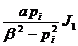§7    Hankel Transform

[ Hankel transform and its inversion formula ]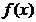The v -order Hankel transform of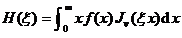The inversion formula of the v -order Hankel transform is: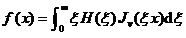where J v ( x ) is a Bessel function .

[ Hankel transformation table ]v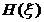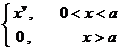>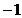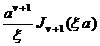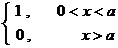0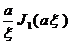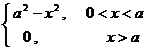0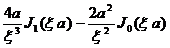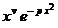>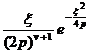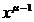>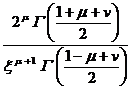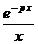0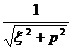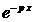0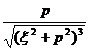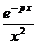1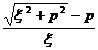1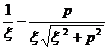1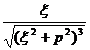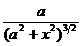0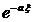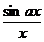0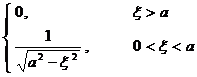1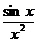0[ Finite Hankel transform and its inversion formula ]The finite Hankel transform of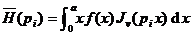where pi is a root of the equation .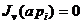The inversion formula of the finite Hankel transform is: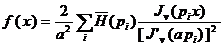where is the sum of all positive roots of the equation .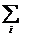[ Finite Hankel transformation table ]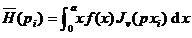where is the sum of all positive roots of the equation .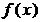v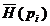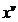>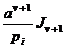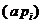c 0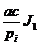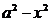0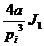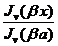>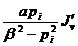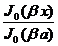0